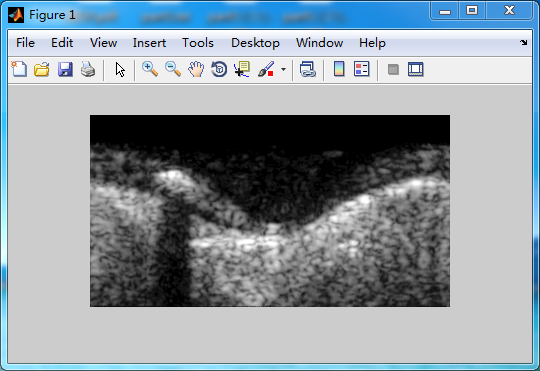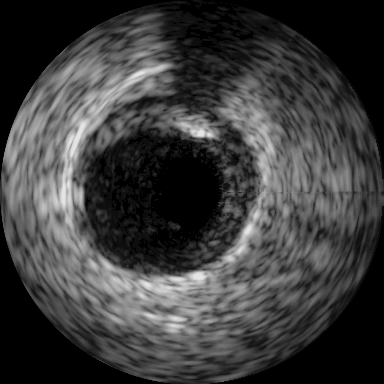function pim = polartrans1(im, nrad, ntheta, cx, cy, linlog, shape)
[rows, cols] = size(im);
if strcmp(shape,'valid') % Find minimum radius value
rmax = min([cx-1, cols-cx, cy-1, rows-cy]);
end
deltatheta = 2*pi/ntheta;

if strcmp(linlog,'linear')
end
xi = radius.*cos(theta) + (rows+1)/2; % Locations in image to interpolate data
yi = -radius.*sin(theta) + (cols+1)/2; % y轴方向与矩阵方向相反

[x,y] = meshgrid([1:cols],[1:rows]);
pim = interp2(x, y, double(im), xi, yi,'linear',0);

3个回答huntermjy123 我也找过这个代码，不过还是拿不到想要的图像，可以使用自带的函数pol2cart来做吗
5 年多之前 回复

https://www.cnblogs.com/tiandsp/archive/2013/06/09/3129198.html